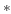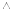TraubsHHNeuron
Subsections

## TraubsHHNeuron

This model is a modified version of Traub's model of Na/K currents for action potentials in hippocampal pyramidal cells, which was modified from HH equations to fit the kinetics of those neurons; the rate constants correspond to a temperature of 36 deg C

The model is based on a CbNeuron and includes the Traubs_HH_K_Channel and Traubs_HH_Na_Channel for action potetial generation.

INa = gNam3h(v-ENa) IKd = gKdn4(v-EK)

m' = (m_inf - m) / tau_m h' = (h_inf - h) / tau_h n' = (n_inf - n) / tau_n

v2 = v - Vtr

alpha_m = 0.32(13-v2) / ( exp((13-v2)/4) - 1) beta_m = 0.28(v2-40) / ( exp((v2-40)/5) - 1) tau_m = 1 / (alpha_m + beta_m) m_inf = alpha_m / (alpha_m + beta_m)

alpha_h = 0.128exp((17-v2)/18) beta_h = 4 / ( 1 + exp((40-v2)/5) ) tau_h = 1 / (alpha_h + beta_h) h_inf = alpha_h / (alpha_h + beta_h)

alpha_n = 0.032(15-v2) / ( exp((15-v2)/5) - 1) beta_n = 0.5exp((10-v2)/40) tau_n = 1 / (alpha_n + beta_n) n_inf = alpha_n / (alpha_n + beta_n)

HH parameters:

gNa = 0.1 S/cm2 gKd = 0.03 S/cm2 ENa = 50 mV EK = -90 mV Vtr = -63 mV (adjusts threshold to around -50 mV)

Vthresh (V) :
If Vm exceeds Vthresh a spike is emmited.
Vreset (V) :
The voltage to reset Vm to after a spike.
doReset (flag) :
Flag which determines wheter Vm should be reseted after a spike
Trefract (sec) :
Length of the absolute refractory period.
nummethod (flag) :
Numerical method for the solution of the differential equation: Exp. Euler = 0, Crank-Nicolson = 1
type :
Type (e.g. inhibitory or excitatory) of the neuron
Cm (F) :
The membrane capacity Cm
Rm (Ohm) :
The membrane resistance Rm
Vresting (V) :
The resting membrane voltage.
Vinit (V) :
Initial condition forVm at time t=0.
VmScale (V) :
Defines the difference between Vresting and the Vthresh for the calculation of the iongate tables and the ionbuffer Erev.
Inoise (W2) :
Variance of the noise to be added each integration time constant.
Iinject (A) :
Constant current to be injected into the CB neuron.

Em (V) :
The reversal potential of the leakage channel
Vm (V) :
The membrane voltage
Isyn :
synaptic input current
Gsyn :
synaptic input conductance
nIncoming :
Number of incoming synapses
nOutgoing :
Number of outgoing synapses
nBuffers :
Number of ion buffers
nChannels :
Number of channels